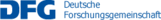## A02 –  The quantum Boltzmann equation

The dynamics of many weakly interacting quantum particles can be described by the so-called Boltzmann-Nordheim equation. This is a kinetic equation, which describes the evolution of the phase space densities of the system. This equation is the quantum counterpart of the classical Boltzmann equation. The aim of this project is to obtain analytic information on the behaviour of the solutions of this equation.

There are two different forms of the Boltzmann-Nordheim equation, for bosonic and fermionic particles respectively. We are mostly interested in the first case, where the stationary solutions of the Boltzmann-Nordheim equation are the well known Bose-Einstein distributions. At sufficiently low temperature, these distributions have a macroscopic number of particles in the state of zero energy. This phenomenon is known as Bose-Einstein condensation.

The standard derivations of the Boltzmann-Nordheim equation in the classical literature do not take the presence of the condensate into account. However, this equation predicts the formation of the condensate. Actually the onset of the condensate emerges in a natural manner as a consequence of the study of the dynamics of the solutions of the equation.

In this project we intend to analyse rigorously the onset of the condensation phenomenon. This includes establishing the possible onset of singularities in finite time, as well as studying the effect of specific type of regularizations used for the collision kernel on the dynamics of weak solutions.

Name Institute Location Phone
Velázquez, Juan JoséIAMEn60/2.02362378velazquez@iam.uni-bonn.de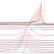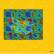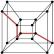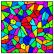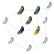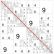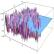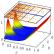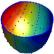## Contact

Coordinator: Prof. Dr. Stefan Müller